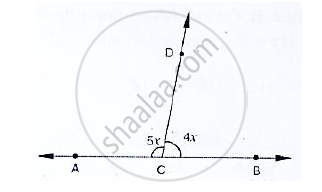# In the Below Fig, Acb is a Line Such that ∠Dca = 5x and ∠Dcb = 4x. Find the Value of X. - Mathematics

In the below fig, ACB is a line such that ∠DCA = 5x and ∠DCB = 4x. Find the value of x.#### Solution

Here, ∠ACD + ∠BCD = 180°

[Since ∠ACD, ∠BCD are linear pairs]

∠ACD = 5x, ∠BCD = 4x

⇒  5x + 4x = 180°

⇒  9x = 180°

⇒ x = 20°

∴x = 20°

Concept: Pairs of Angles
Is there an error in this question or solution?
Chapter 10: Lines and Angles - Exercise 10.2 [Page 15]

#### APPEARS IN

RD Sharma Mathematics for Class 9
Chapter 10 Lines and Angles
Exercise 10.2 | Q 11 | Page 15

Share